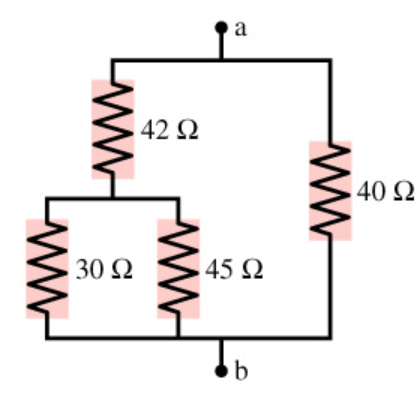# Problem: What is the equivalent resistance between points a and b in the figure?

###### FREE Expert Solution

Resistors in parallel:

$\overline{){{\mathbf{R}}}_{\mathbf{e}\mathbf{q}}{\mathbf{=}}\frac{{\mathbf{R}}_{\mathbf{1}}{\mathbf{R}}_{\mathbf{2}}}{{\mathbf{R}}_{\mathbf{1}}\mathbf{+}{\mathbf{R}}_{\mathbf{2}}}}$

Resistors in series:

$\overline{){{\mathbf{R}}}_{\mathbf{e}\mathbf{q}}{\mathbf{=}}{{\mathbf{R}}}_{{\mathbf{1}}}{\mathbf{+}}{{\mathbf{R}}}_{{\mathbf{2}}}}$

30Ω and 45Ω resistors are in parallel:

Req = (30)(45)/30+45 = 18Ω###### Problem DetailsWhat is the equivalent resistance between points a and b in the figure?